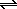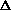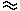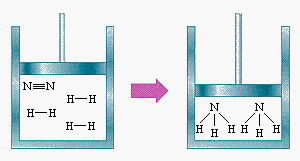Le Chatelier's Principle

Le Chatelier's Principle

 Le Chatelier's Principle Changes in Concentration Changes in Pressure Changes in Temperature

Le Chatelier's Principle

In 1884 the French chemist and engineer Henry-Louis Le Chatelier proposed one of the central concepts of chemical equilibria. Le Chatelier's principle can be stated as follows: A change in one of the variables that describe a system at equilibrium produces a shift in the position of the equilibrium that counteracts the effect of this change.

Le Chatelier's principle describes what happens to a system when something momentarily takes it away from equilibrium. This section focuses on three ways in which we can change the conditions of a chemical reaction at equilibrium:

(1) changing the concentration of one of the components of the reaction

(2) changing the pressure on the system

(3) changing the temperature at which the reaction is run.Changes in Concentration

To illustrate what happens when we change the concentration of one of the reactants or products of a reaction at equilibrium, let's consider the following system at 500oC.

 N2(g) + 3 H2(g)2 NH3(g) Kc = 0.040 Initial: 0.100 M 0.100 M 0 Equilibrium: 0.100 -C 0.100 - 3C 2C

We obtain the following results when we solve this problem.

 [NH3] = 2C0.0020 M [N2] = 0.100 -C0.099 M [H2] = 0.100 - 3C0.097 M

The fact thatC is small compared with the initial concentrations of N2 and H2 makes this calculation relatively easy to do. But it implies that very little ammonia is actually produced in the reaction. According to this calculation, only 1% of the nitrogen present initially is converted into ammonia.

What would happen if we add enough N2 to increase the initial concentration by a factor of 10? The reaction can't be at equilibrium any more because there is far too much N2 in the system. Adding an excess of one of the reactants therefore places a stress on the system. The system responds by minimizing the effect of this stressby shifting the equilibrium toward the products. The reaction comes back to equilibrium when the concentrations of the three components reach the following values.

 [NH3] = 2C0.0055 M [N2] = 1.00 -C1.00 M [H2] = 0.10 - 3C0.092 M

By comparing the new equilibrium concentrations with those obtained before excess N2 was added to the system, we can see the magnitude of the effect of adding the excess N2.

 Before After [NH3]0.0094 M [NH3]0.026 M [N2]0.095 M [N2]0.99 M [H2]0.29 M [H2]0.26 M

Increasing the amount of N2 in the system by a factor of 10 leads to an increase in the amount of NH3 at equilibrium by a factor of about 3. Adding an excess of one of the products would have the opposite effect; it would shift the equilibrium toward the reactants.Changes in Pressure

The effect of changing the pressure on a gas-phase reaction depends on the stoichiometry of the reaction. We can demonstrate this by looking at the result of compressing the following reaction at equilibrium.

 N2(g) + 3 H2(g)2 NH3(g)

Let's start with a system that initially contains 2.5 atm of N2 and 7.5 atm of H2 at 500oC, where Kp is 1.4 x 10-5, allow the reaction to come to equilibrium, and then compress the system by a factor of 10. When this is done, we get the following results.

 Before Compression After Compression PNH3 = 0.12 atm PNH3 = 8.4 atm PN2 = 2.4 atm PN2 = 21 atm PH2 = 7.3 atm PH2 = 62 atm

Before the system was compressed, the partial pressure of NH3 was only about 1% of the total pressure. After the system is compressed, the partial pressure of NH3 is almost 10% of the total.

These data provide another example of Le Chatelier's principle. A reaction at equilibrium was subjected to a stressan increase in the total pressure on the system. The reaction then shifted in the direction that minimized the effect of this stress. The reaction shifted toward the products because this reduces the number of particles in the gas, thereby decreasing the total pressure on the system, as shown in the figure below.N2(g) + 3 H2(g)2 NH3(g)Changes in Temperature

Changes in the concentrations of the reactants or products of a reaction shift the position of the equilibrium, but do not change the equilibrium constant for the reaction.

Similarly, a change in the pressure on a gas-phase reaction shifts the position of the equilibrium without changing the magnitude of the equilibrium constant. Changes in the temperature of the system, however, affect the position of the equilibrium by changing the magnitude of the equilibrium constant for the reaction.

Chemical reactions either give off heat to their surroundings or absorb heat from their surroundings. If we consider heat to be one of the reactants or products of a reaction, we can understand the effect of changes in temperature on the equilibrium. Increasing the temperature of a reaction that gives off heat is the same as adding more of one of the products of the reaction. It places a stress on the reaction, which must be alleviated by converting some of the products back to reactants.

The reaction in which NO2 dimerizes to form N2O4 provides an example of the effect of changes in temperature on the equilibrium constant for a reaction. This reaction is exothermic.

 2 NO2(g)N2O4(g)Ho = -57.20 kJ

Thus, raising the temperature of this system is equivalent to adding excess product to the system. The equilibrium constant therefore decreases with increasing temperature.

 Practice Problem 7:Predict the effect of the following changes on the reaction in which SO3 decomposes to form SO2 and O2. 2 SO3(g)2 SO2 (g) + O2 (g)Ho = 197.78 kJ (a) Increasing the temperature of the reaction. (b) Increasing the pressure on the reaction. (c) Adding more O2 when the reaction is at equilibrium. (d) Removing O2 from the system when the reaction is at equilibrium. Click here to check your answer to Practice Problem 7